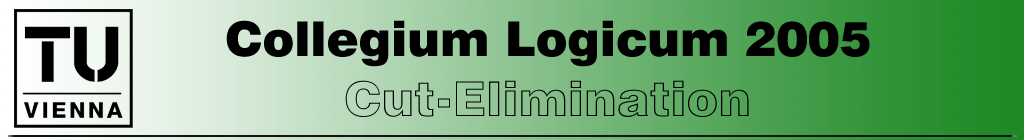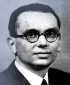Home

Page Top

Arnold Beckmann - "Cut-reduction by switching"

Abstract:

 Usual cut-elimination procedures like the Gentzen- or Tait-style cut elimination methods have the cost of a (super-) exponential increase of the size of derivations. Cut-reduction by switching utilises methods from Boolean complexity to reduce the complexity of cuts in a derivation without increasing the size of the derivation. However, the disadvantage is that not only is the complexity of cut formulas reduced, but also the complexity of the derived formula. In this talk I will explain Cut-reduction by switching and the use of Sipser functions to rescue the derived formula. I will point out applications of cut-reduction by switching, which will mainly be in the area of propositional proof systems . Jan Krajicek  was the first one who used such methods from Boolean complexity in this setting.  J.Krajicek: Lower bounds to the size of constant-depth      Frege proofs. JSL, 59:73-86, 1994.  A.Beckmann and S.Buss: Separation results for the size of      constant-depth propositional proofs. To appear in APAL.

Lev Beklemishev - "On Schmerl's reduction formula and its relation to cut-elimination"

Abstract:

 Schmerl's reduction formula states that, in the context of formal arithmetic, a reflection principle of logical complexity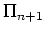can be conservatively approximated by finitely iterated reflection principles of complexity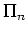. It can be seen as an analog of the cut-elimination theorem for omega-logic. However, there are some notable differences. In the talk we shall compare the two results.

Agata Ciabattoni - "Towards a semantic characterization of cut-elimination"
(joint work with. K. Terui)

Abstract:

 We introduce necessary and sufficient conditions for a (single-conclusion) sequent calculus to admit cut-elimination. Our conditions are formulated both syntactically and semantically.

Rosalie Iemhoff - "Truth value logics and the existence predicate"

Abstract:

 We introduce Scott logics, which are generalizations of Gödel logics that correspond in the same manner to the logics of linear frames as Gödel logics correspond to the logics of linear frames with constant domains. This correspondence result is based on previous results by A. Beckamnn and N. Preining. We discuss the relation to the existence predicate and show that this predicate enables us to define a faithful translation of Scott logics into Gödel logics.

George Metcalfe - "Density Elimination"

Abstract:

 The following density rule was introduced by Takeuti and Titani in the context of intuitionistic fuzzy logic, also known as standard first-order Gödel logic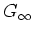: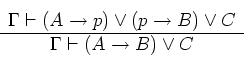where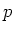is a propositional variable not occurring in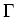,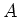,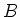, or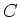. A syntactic elimination of this rule forwas obtained by Baaz and Zach by showing that applications of the corresponding rule in a Gentzen-style hypersequent calculus forcan be shifted upwards in proofs and removed. In this work we establish density elimination for a number of other logics presented in the hypersequent framework, and, as a consequence, provide syntactic proofs of purely algebraic results; namely, that certain varieties are generated by their linearly ordered dense members.

Grigori Mints - "Cut elimination for provability logic"

Abstract:

 Provability logic is one of the most popular modal logics. It is sound and complete for the interpretation of modality as provabiulity in Peano Arithmetic and many other theories. It turns out that there is no accepted syntactic cut elimination (normalization) proof for a standard sequent formulation of the provability logic. A proof by D. Leivant in  contained a gap, subsequent proof by S. Valentini  was also incomplete. A model-theoretic proof of the normal form theorem along the familiar lines was given by several authors. We present a syntactic cut elimination proof along the standard lines. The main step absent in previous papers is the cut-free admissibility of the rule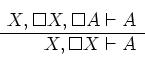Leivant.  Valentini.

Georg Moser - "Ackermann's Substitution Method (remixed)"

Abstract:

 A fascinating result in proof theory is the classification of the provable recursive functions of Peano Arithmetic in terms of Kreisel's class of ordinal recursive functions. This class can in turn be characterised by hierarchies of number-theoretic functions defined by transfinite recursion upto the ordinal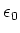. The standard proof of this result employs cut-elimination in a suitably defined semi-formal system. The solution suggested by Kreisel was based on Ackermann's consistency proof of Peano Arithmetic, framed in Hilbert's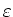-calculus. We present a modern treatment of Ackermann's consistency proof that renders a classification of the provable recursive functions of Peano Arithmetic in terms of the fast-growing Hardy hierarchy upto.

Pavel Pudlák - "On the structure of terms in Herbrand's disjunctions"

Abstract:

 The basic information concerning Herbrand's disjunctions is in which terms which variables occur. This structure determines whether, given a disjunction of term-instances of an open formula, one can derive a first order sentence with a particular quantifier prefix. We shall study this dependence using a two player game with players corresponding to the two quantifiers. Then the structure is determined by the ordering of the moves of the two players. The main problem that we address in the lecture is to find a class of orderings which is as small as possible and such that all logically valid sentences of a given quantifier prefix have Herbrand proofs with such orderings.

Clemens Richter - "Cut-Elimination: Experiments with CERES"

Abstract:

 Cut-elimination is the most prominent form of proof transformation in logic. The elimination of cuts in formal proofs corresponds to the removal of intermediate statements (lemmas) in mathematical proofs. The cut-elimination method CERES (cut-elimination by resolution) works by constructing a set of clauses from a proof with cuts. Any resolution refutation of this set can then serve as a skeleton of a proof with only atomic cuts. In this talk we present an implementation of CERES, in particular the basic algorithms, the input format and the integration of the well-known automated theorem prover 'Otter'. Furthermore a systematic experiment with the implementation of CERES on a proof of reasonable size and complexity will be presented. It turns out that the proof with cuts can be transformed into two "mathematically" different proofs of the theorem. In particular, the application of positive and negative hyperresolution yield different mathematical arguments. As an unexpected side-effect the derived clauses of the resolution refutation proved particularly interesting as they can be considered as meaningful universal lemmas. Though the proof under investigation is intuitively simple, the experiment demonstrates that new (and relevant) mathematical information on proofs can be obtained by computational methods. It can be considered as a first step in the development of an experimental culture of "computer-aided proof analysis" in mathematics.

Helmut Schwichtenberg - "Program Extraction from Normalization Proofs"
(joint work with Ulrich Berger and Pierre Letouzey)

Abstract:

 We formalize Tait's proof of the existence of normal forms for terms of the simply typed lambda-calculus, and machine-extract a program from this proof, which turns out to implement the well-known normalization by evaluation algorithm. On paper, this has already been done by Ulrich Berger (TLCA 1993). However -- as is to be expected -- the formalization turned out to be not at all a trivial matter, so that it appears to be worthwhile to describe some of the choices which have simplified the task considerably. On the other hand, a full formalization is necessary for machine extraction of a program from such a proof. We carry this out for the proof assistant Minlog which has a suitable program extraction machinery built in. This provides a useful occasion to test this machinery in a non-trivial setting.

Christian Urban - "On the Cambridge Interpretation of Cut-Elimination in Classical Logic"

Abstract:

 It is a familiar idea that in intuitionistic logic one can interpret the process of normalisation, and hence also cut-elimination, as computation in the lambda-calculus. There have been many proposals to obtain a similar interpretation for nomalisation and cut-elimination in classical logic. In the talk I will give an overview about the ideas a group of people had in Cambridge concerning the computational content of classical proofs. The starting point for this overview is a strongly normalising cut-elimination procedure for classical logic. This cut-elimination procedure provides a unifying framework in which the existing proposals can be embedded and in which one can experiment with many phenomena of cut-elimination in classical logic. I will discuss how far we have come in Cambridge and where we failed to provide clear cut results.